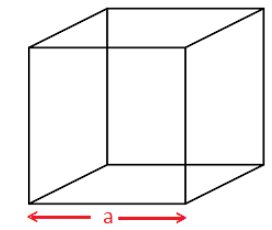# Surface Area of a Cube Formula

## Definition

Surface Area of a cube is the total area of the outside surfaces of the cube and is given by A= 6a2, where a is the edge.

A cube has 6 identical square faces and hence it is also called as a hexahedron. Each face of a cube has 4 edges and totally there are 12 edges. It is measured in terms of square unit.### How to Find the Surface Area of a Cube

The Surface Area of a Cube Formula is,

 Surface area of Cube = 6a2, where “a” is the side length of the cube.

### Solved Examples

Question 1: What is the surface area of a cube of side 5 cm?

Solution:

Given,
Side of the cube = a = 5 cm
Surface Area of a Cube = 6a2
= 6 × 52 cm2
= 6 × 25 cm2
= 150 cm2
Question 2: What is the surface area of a cube of side 17 cm?

Solution:

Given,
Side of the cube = a = 17 cm
Surface Area of a Cube = 6a2
= 6 × 172 cm2
= 6 × 289 cm2
= 1734 cm2

Question 3: Find the side of a cube whose surface area is 1014 cm2.

Solution:

Given surface area of a cube = 1014 cm2

Let “a” be the side of the cube.
We know that,

Surface area of cube = 6a2

⇒ 6a2 = 1014

⇒ a2 = 1014/6

⇒ a2 = 169

⇒ a = √169

⇒ a = 13

Therefore, side of the cube = 13 cm